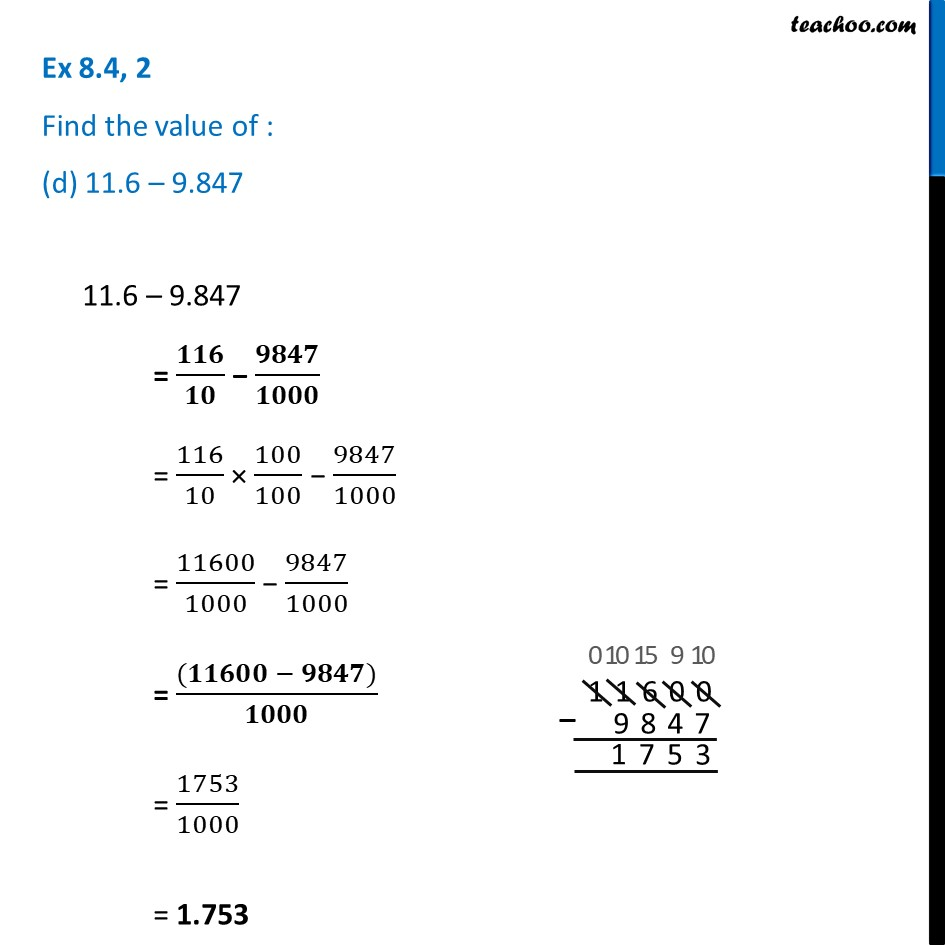Ex 8.4

Chapter 8 Class 6 Decimals
Serial order wiseLearn in your speed, with individual attention - Teachoo Maths 1-on-1 Class

### Transcript

Ex 8.4, 2 Find the value of : (d) 11.6 – 9.84711.6 – 9.847 = 𝟏𝟏𝟔/𝟏𝟎 − 𝟗𝟖𝟒𝟕/𝟏𝟎𝟎𝟎 = 116/10 × 100/100 − 9847/1000 = 11600/1000 − 9847/1000 = ((𝟏𝟏𝟔𝟎𝟎 − 𝟗𝟖𝟒𝟕))/𝟏𝟎𝟎𝟎 = 1753/1000 = 1.753